Next: Criticism of the relativistic cosmology Up: Criticism of the GRT basis Previous: Time in GRT   Contents

## Some GRT corollaries

Now we pass to mathematical methods of GRT and to corollaries of this theory. The games with the space-time properties result in the fact, that in GRT the application of variation methods occurs to be questionable: the quantities are not additive, the Lorentz transformations are non-commutative, the integral quantities depend on the path of integration. Even it is not clear, how the terminal points can be considered as fixed, if the distances are different in different frames of reference.

Because of nonlocalizableness (non-shieldness) of gravitation field, conditions on infinity (because of the mass absence on infinity, it is euclideanness) are principally important for the existence of the conservation laws in GRT  (for systems of the insular type only). The classical approach is more successive and useful (theoretically and practically): energy is determined correctly to a constant, since the local energy difference between two transition points has a physical meaning only. Therefore, conditions on infinity is groundless.

Highly doubtful is the procedure of linearization in the general form, since it can be only individual. The tending to simplicity is declared, but even two types of time are introduced - coordinate and intrinsic time. The fitting to the well-known or intuitive (classically) result is often made. So, for motion of Mercury's perihelion  the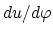derivative can have two signs. Which of them should be chosen? To say already nothing of the fact, that the dividing byis performed, but this quantity can be zero. The complexity of spatial-temporal links is stated, but eventually one passes for a very long time to customary mathematical coordinates; otherwise there is nothing to compare the results with. For what was there a scrambling? For pseudo-scienceness?

Till now there is no sufficient experimental proof of whether the rate of transmission of gravitational interactions is higher than, lower than, or exactly equal to, light speed (as is postulated in GRT). For example, Laplace and Poincare believed on the basis of observations [24,87], that the rate of transmission of gravitational interactions is several orders greater than the light speed.

Now we note on the experimental substantiation of the GRT. Usually, even there exists a hundred different data, a theory is constructed not always: the data can simply be tabulated in a table. But in the case of the GRT we see "the Great theory of three and half observations", three of which are the fiction. Concerning the light deflection from rectilinear motion in a gravitational field, we should make the following statements. First, as it was pointed out by many experimentalists, a quantitative verification of an effect essentially depends on the faith of the concrete experimentalist. Second, even from the classical formula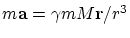it follows that any "object" (even of zero or negative mass) will "fall down" in the gravitational field. Third, with which a value does the effect be compared? With a value in empty space? As early as 1962, a group of Royal astronomers declares that the light deflection near the Sun cannot be considered as confirmation of GRT, because the Sun has an atmosphere stretching for a great distance. We would remind that the effect of refraction is long taken into account by astronomers for the terrestrial atmosphere. Lomonosov discovers the deflection of a light beam in the atmosphere of the Venus long ago. For explanation, imagine a glass sphere. Naturally, parallel rays (from distant stars) will be deflected to the center in it. Such a system is well known as an optical lens. The similar situation will take place for a gas sphere (the Sun's atmosphere). For accurate calculation of light beam deflection in the gravitational field, one should take into account the presence of the solar atmosphere and the fact, that density and temperature gradients on the beam path causes changes to the medium's refractive index and, hence, to the bending of the light beam. Even at the distance of a hundred of meters (near the Earth), these effects cause a mirage, so ignoring them for a beam coming from a star and passing near the Sun (at millions of kilometers) is a pure speculation.

The displacement of the perihelion of Mercury is, of course, a remarkable effect (but whether the sole example is sufficient "to attract" a scientific theory, or not?). Therefore, it would be interesting to observe it near solid bodies (for the satellite of the planets, for instance), so that the value of this effect could be estimated for certainty. The matter is that the Sun is not a solid body, and the motion of Mercury may cause a tidal wave on the Sun, which may in turn also cause a displacement of Mercury's perihelion. (Depending on the rate of transmission of gravitational interactions and "hydrodynamic" properties of the Sun, the tidal wave may either outstrip, or lag behind the motion of Mercury.) In any case, it is necessary to know the rate of transmission of gravitational interactions for calculating the effect of a tide due to the Mercury and other planets on Mercury's orbit characteristics, in order that the purely "gravitational" effect (if it exists) of the general relativity theory could be separated.

Calculating the perihelion displacement in GRT (from the rigorous solution for a single attractive point), the impression is given that we know astronomical masses exactly. If we use GRT as a correction to Newton's theory, the situation is in fact opposite: there exists a problem knowing visible planet motions to reestablish the exact planet masses (to substitute the latters and to check GRT thereafter). Imagine the circular planet orbit. It is obvious in this case, that the Newtonian rotation period will already be taken with regard to an invisible precession, i.e. the period will be renormalized. Therefore, renormalized masses of planets are already included in Newton's gravitation theory. Since the GRT-corrections are much less than the perturbation planet actions and the influence of a non-sphericity, the reestablishment of exact masses can essentially change the description of a picture of the motion for this complex many-body problem. No such detailed analysis was carried out. Generally speaking, the situation with description of the displacement of the Mercury's perihelion is typical for relativist's behaviour. First, it was declared that the effect was predicted, but Einstein compares it with the well known results of approximate calculations, which was produced by Laplace long before origin of the GRT. Hope, each man understands a great difference between "predict" and "explain after the event" (remember the appropriate anecdote of Feynman). Second, there exists the most part of precession already in classical physics: the data of 19th century was found with taking into account influences of some planets. The result obtained was the value of 588'', whereas a deficiency in the calculated value make up about 43'' only, that is a small correction. (Note, that some data of 20th century indicate the total value of precession to be about 10 times higher than mentioned one, but the "deficiency for GRT" in 43'' is maintained - "taboo"; nevetheless, it could be a misprint and we will not cavil to 1/3 of "the great experimental base of GRT"). Third, the exact calculation for a many-body problem cannot yet be made even by the modern mathematics. In classical case the calculation was made as a sum of independent corrections from influences of separate planets (the Sun and planets were considered as material points). Naturally, the classical net result (more than 90% from observable one!) can some more be improved with taking into account the solar non-sphericity, influences of all planets (including small bodies) of the solar system, the fact that the Sun is not a solid object (a material point) and its local density in different layers must "follow" influences of other moving planets. Most probably, this way of using real physical mechanisms can lead to obtaining the deficient small effect. But the relativist's declaration is inconceivable speculation! They "found" an effect (the small procent only) considering motions of two material points only - the Sun and the Mercury. Sorry, and what will a correction be made with the GRT for the most part of the effect obtained classically? Do you fear to calculate? Then on what "a brilliant coincidence" do you repeat? It is the pure machination to a desired result!

The prototype of the "black hole" in Laplac's solution, where the light, moving parallel to the surface, begins to move over a circle like the artificial satellite of the Earth, differs from the GRT ideas. Nothing prohibits the light with a rather high energy to escape the body in the direction perpendicular to its surface. There is no doubt, that such beams will exist (both by internal and external reasons): for example, the beams falling from outside will be able to accumulate energy, in accordance with the energy conservation law, and to leave such a "black hole" after reflecting. Instead of invoking contradictory properties of light, we simply consider the "fall" of an elementary particle - an electron, for example. Whether the possibility of the elastic reflection is maintained for it, or such the possibility must postulatively be forbidden (to rescue the GRT)? And if such the possibility is not forbidden, then we consider the following process. Let an electron be coming into fall with the zero start velocity from a distant point A (at the distance 100 a.u., for example) to a very massive body (Fig. 2.8).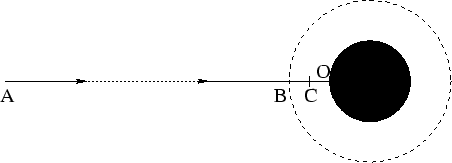The body absorbs "last surplus nearest molecules" and becomes the "black hole" in a matter of an instant before the electron crosses the Schwarzschild sphere (which is marked as B on the picture). To be visual, the distance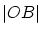is shown comparatively large. In a matter of an instant before the collision of the electron with the surface of the "black hole" the latter object was stable, and since neither velocity nor acceleration of this surface can instantly become very large (besides, the collision can take place with a particle flying to meet), then at the elastic collision the electron will fly to the point A with just the same speed as it acquires before the collision. Relativists claim that it cannot get over the Schwarzschild sphere B. Let it come to a stop at the point C (at the distance 10 km from the body center, for example). If the energy conservation law is obeyed, and since the electron's velocity equals to zero at the points A and C, then the potential energy of the electron at the point A is equal to the potential energy at the point C. Therefore, the gravitational field (attractive forces) is absent between the points A and C, or else the potential might be monotonically decreasing. However, the consideration of the situation from the pure GRT positions leads to the still worse result (see below). The "black holes" in GRT is a real mysticism. If we take a long rod, then at motion its mass will increase and the size will decrease (according to SRT). What will happen? Is the "black hole" generated? All the sky will become filled with "black holes", if we shall move rapidly enough. And, you see, this process would be irreversible in GRT. For example, any object of the Universe is a "black hole" for fast moving light (how it can exist?).

Recall some well-known solutions: 1) the Schwarzschild solution describes the centrally symmetric "field" in vacuum (note that the temperature characteristic is absent, i.e.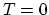K); and 2) the axially symmetric Kerr's metric describes the "field" of a rotating collapsing body. The presence of singularities or multiple connection of the solution implies, that, as a minimum, the solution is inapplicable in these regions. Such a situation takes place with the change of the space - time signature for the "black hole" in the Schwarzschild solution, and it is not necessary to search any artificial philosophical sense in this situation. The singularity in the Schwarzschild solution for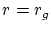cannot be eliminated by purely mathematical manipulations: the addition of the infinity with the other sign at this point is the artificial game with the infinities, but such a procedure requires the physical basis. (You see, in physics all singularities at zero are not eliminated by artificial addition of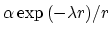, where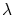is a large quantity).

Even from GRT follows the impossibility of observation of "black holes": the time of the "black hole" formation will be infinite for us as remote observers. And since the collapse cannot be completed, the solutions, which consider all things as though they have already happened, have no sense (even if we were waited till "the End of the World", no one "black hole" could have time to form). The separation of events by infinite time for internal and external observers is not "an extreme example of the relativity of the time course", but the elementary manifestation of the inconsistency of Schwarzschild's solution. The same fact follows from the "incompleteness" of systems of solutions. It is not clear, what will happen with the charge conservation law, if a greater quantity of charges of the same sign will enter the "black hole"? The mystical description of "metrical tidal forces"  at approaching the "black hole" is invalid, since it would mean, that the gravitation force gradient is great within the limits of a body, but all GRT ideas are based on the opposite assumptions. The Kerr metric in the presence of rotation also clearly demonstrates the inconsistency of GRT: it gives in a strict mathematical manner several physically unreal solutions (the same operations, as for Schwarzschild's metric, do not save the situation). Thus, such the GRT objects as the "black holes" cannot exist and they must be transfered from the realm of sciences to the province of the non-scientific fiction. All the Universe is evidence of the wonderful (frequently dynamical) stability: there do not exist infinite collapses (an explosion can happen sooner). All this does not cancel a possibility of the existence of superheavy (but dynamically stable) objects which can really be manifested by several effects (for example, by accretion, radiation etc.). No the GRT fabrications are required for these purposes at all. We have no need to seek ways for the artificial rescue of the GRT, such as the "evaporation of the black holes", since such a possibility is strictly absent in the GRT (the speed of light cannot be overcome). On the contrary, in classical physics no problems exist at all.

GRT contains a lot of doubtful prerequisites and results. List some of them. For example, the requirement of gravitational field weakness for low velocities is doubtful: if the spacecraft is landed on a massive planet, whether it can not stand or slowly move? Whether some molecules with low velocities cannot be found in spite of temperature fluctuations? The consideration of a centrally symmetric field in GRT has not physical sense as well: since the velocity can be only radial, then not only rotations, but even real temperature characteristics can not exist (i.e.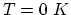). The field in a cavity is not obtained in a single manner, but, simply, two various constants are postulated in order to avoid singularities.

The emission of gravitation waves for a parabolic motion (with eccentricity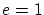) results in the infinite loss of energy and angular momentum, which obviously contradicts the experimental data.

In fact, GRT can be applied only for weak fields and weak rotations, i.e. in the same region, as the Newtonian theory of gravitation. Recall that the similar interaction between moving charges differs from the static Coulomb law. Therefore, prior to applying the static Newtonian law of gravitation, it must be verified for moving bodies, but this is a prerogative of the experiment.

Let us discuss one more principal point concerning the relativity of all quantities in GRT. The laws, written simply as the equations, determine nothing by themselves. The solution of any problem still requires the knowledge of specific things, such as the characteristics of a body (mass, shape etc.), the initial and/or boundary conditions, the characteristics of forces (magnitude, direction, points of application etc.). The "reference points" are actually specified, with respect to which the subsequent changes of quantities (position, velocity, acceleration etc.) are investigated. The principal relativity of all quantities in GRT contradicts the experiments. The subsequent artificial attempt to derive accelerations (or rotations) with respect to the local geodesic inertial Lorentzian system - this is simply the fitting to only workable and experimentally verified coordinates of the absolute space (GRT does not contain any similar things organically ).

The gravitational constant is not a mathematical constant at all, but it can undergo some variations . Therefore, this value can account corrections to Newton's static law of gravitation (for example, these influences do not taken into consideration for the displacement of the perihelion of the Mercury). We are reminded that in finite moving (periodic, for example) different resonance phenomena can be observed for a coupled many-body system. The effect is manifested in a conforming correction of orbital parameters (especially taking into account a finite size of bodies: non-sphericalness of their form and/or of the mass distribution).

Generally speaking, the theory of short range for gravitation could be useful (but it can be not useful depending on the gravitation transmission rate) for the finite number of cases only: for the rapid (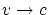) motion of massive (the same order) bodies close to each other. The author does not know such practical examples.

The GRT approach to gravitation is unique: to be shut in the lift (to take pleasure from the fall) and to be not aware that the end (hurt oneself) will be after a moment. Of course, the real state is quite different one: we see always where and how we move relative to the attractive center. Contrary to Taylor and Wheeler, it is the second "particle", together with the first "particle" - with the observer. That is the reason that the pure geometric approach is a temporal zigzag for physics (although it could ever be useful as a auxiliary technique). And two travelers from the parable  (allegedly demonstrate the approach of the geometry of curved space) have need for "very little": for the wish to move from the equator just along meridians (on the spheric earth surface), but the rest of five billion mans can not have such the wish. Contrary to traveler's wish, the wish "to do not attract to the Earth (or the Sun) and to fly away to space" is inadequate. The notion force (the force of gravity in this case) reflects this fact. Geometry cannot answer to the following questions: how many types of interactions exist in nature, why there exist they only, why there exist local masses, charges, particles, why the gravitational force is proportional just to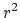, why there realize the specific values of physical constants in nature, and many other questions. These problems are the physical (experimental) prerogative.Next: Criticism of the relativistic cosmology Up: Criticism of the GRT basis Previous: Time in GRT   Contents
Sergey N. Arteha